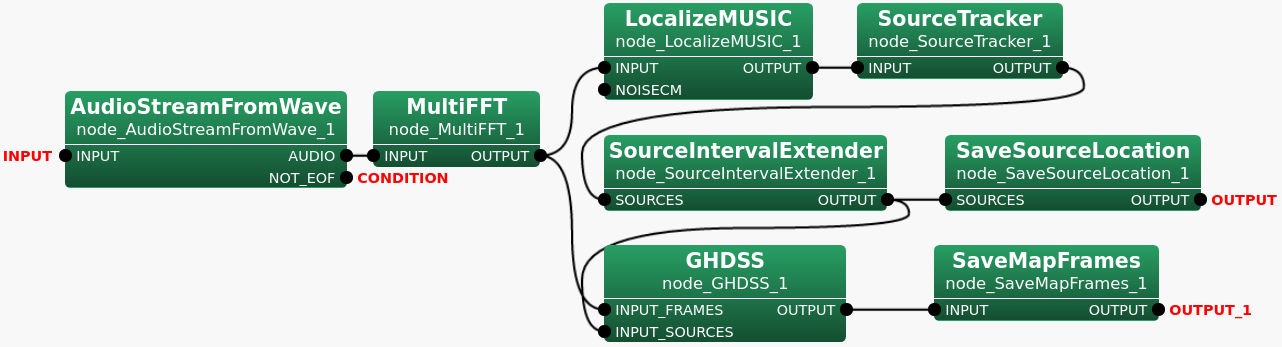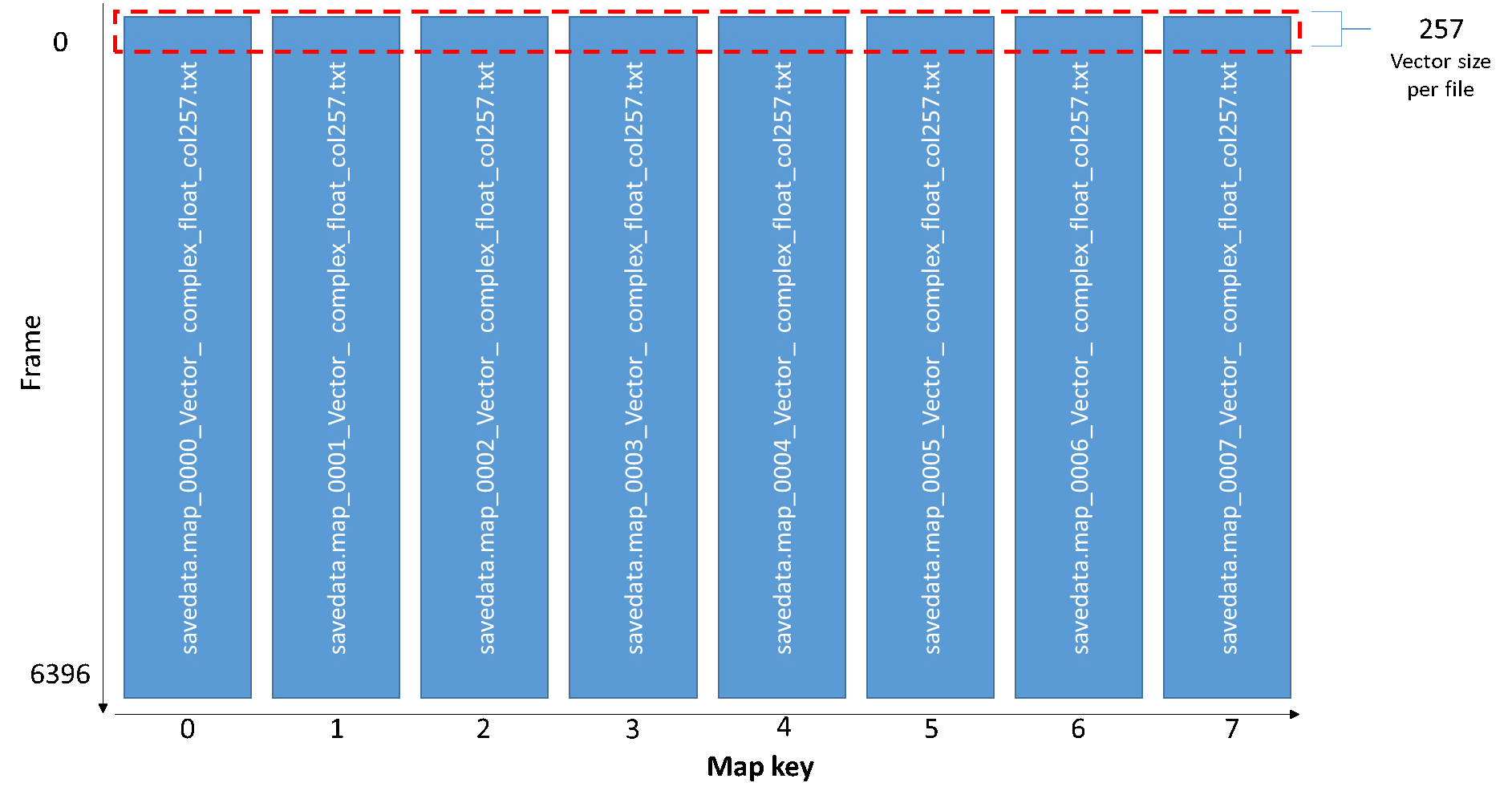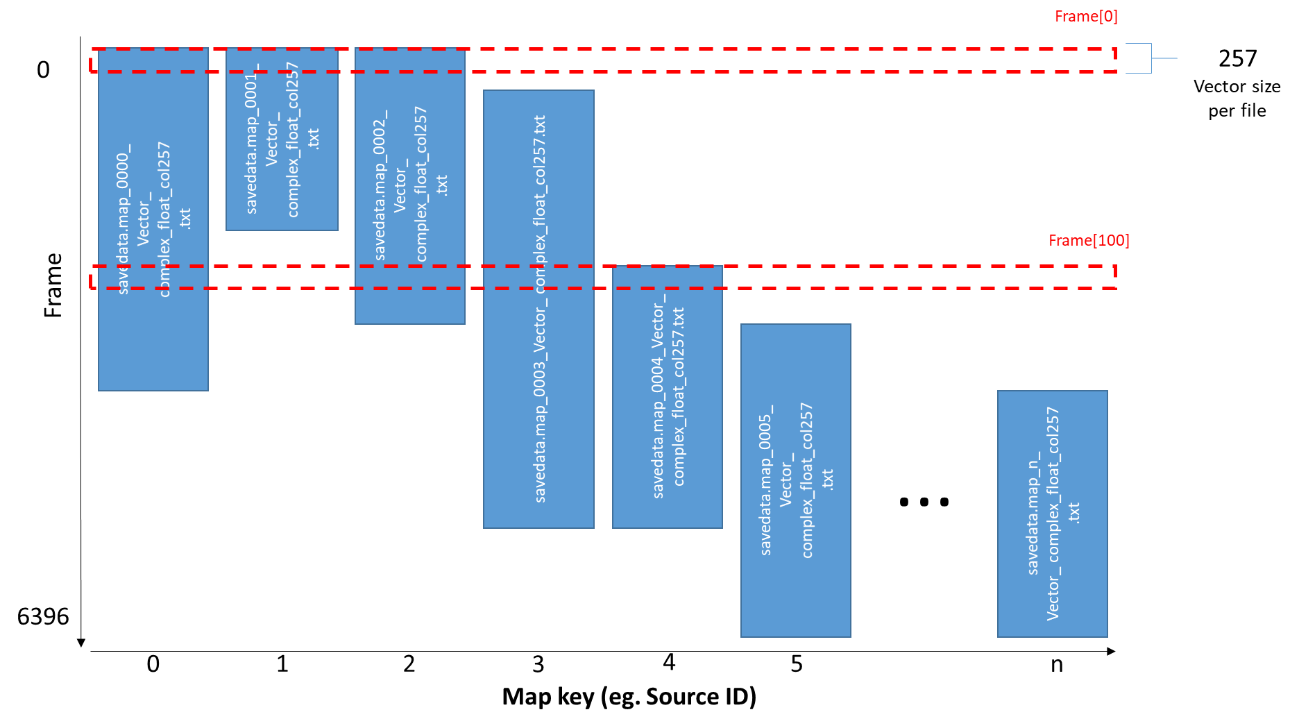6.7.26 SaveMapFrames

6.7.26.1 Outline of the node

Saves data of frames stored in the Map<int, ObjectRef> type of containers in files separately for each key of the Map. Provides a file containing the data file list for each frame in addition to these data files. Note that the supported ObjectRef are the Vector<float> type and the Vector<complex<float> > type.

6.7.26.2 Necessary file

No files are required.

6.7.26.3 Usage

When to use

This node is used to save data of frames separately in a file for each key of the Map<int, ObjectRef> type in which the data of frames originally were stored. Each file generated for each key will consist of data mapped to the same key. In addition, a file containing the lists of these files per frame will be generated. In the example network given in the "Typical connection" below, SaveMapFrames takes data in the Map$<$int , Vector<complex<float> > $>$ type outputted by GHDSS as the input. The data are the pairs of a sound source ID of a separated sound and the complex spectrum of the separated sound. Here, keys are representing sound source IDs. Each file generated for each key will consist of data mapped to the same sound source ID.

Typical connection

Figure 6.129 shows a connection example using SaveMapFrames in a network. In this network, sound source separation is performed using GHDSS algorithm, and the output will be saved in files by SaveMapFrames . For more details on sound separation based on GHDSS algorithm, refer to the node reference of GHDSS .Figure 6.129: Connection example of SaveMapFrames

6.7.26.4 Input-output and property of node

Input

INPUT

: Data of frames in the Map<int, ObjectRef> type where the data are mapped to keys of the Map. Note that the supported types are the Map$<$int , Vector<float> $>$ type and the Map$<$int , Vector<complex<float> > $>$ type.

Output

OUTPUT

: Two types of files. The one is a data file, called "Internal file", and the other one is a list of the "Internal file", called "Main file". The "Internal file" is to be generated as many as the number of keys for the input Map<int, ObjectRef> so the total number of output files will be the number of keys plus 1.

Parameter

Table 6.126: Parameter list of SaveMapFrames
 Parameter name Type Default value Unit Description FILENAME The file name of the text file to output which contains lists of data files per frame. This file name will be a base name of the file name for the data files to output as well. OUTPUTTYPE TEXT The file format options for the data files to output. Select TEXT for text files or RAW for binary files. TEXTFORMAT FIXED The file format options when the OUTPUTTYPE is set to TEXT. Select FIXED or SCIENTIFIC.
FILENAME

: string  type. The file name of the "Main file" in which lists of the "Internal files" for each frame are written.

OUTPUTTYPE

: string  type. The file format options for the "Internal file". Select TEXT for text files or RAW for binary files. The default value is TEXT. Note that the "Main file" is a text file regardless of the values specified in this parameter.

TEXTFORMAT

: string  type. The file format options when the OUTPUTTYPE is set to TEXT. Select FIXED or SCIENTIFIC.

6.7.26.5 Details of the node

SaveMapFrames receives data of frames stored in either the Map$<$int , Vector<float> $>$ type or the Map$<$int , Vector<complex<float> > $>$ type of containers for each frame as the input. Then it saves the data in separate files for each key. The output will be two types of files as follows.

Two types of output files:

1. Main file – A text file in which the "Internal files (see below)" lists for all frames are written. Each list is separated per frame.

2. Internal files – Data files in which data of frames in the Map<int, ObjectRef> type were saved separately for each key. These files will be created as many as the number of the keys. Each file consists of frame data mapped to the same key. The file format is to be specified in the OUTPUTTYPE parameter. When the OUTPUTTYPE parameter is set to RAW, data of frames will be written in IEEE 754 32-bit single-precision floating-point number format by little- endian order. The default value of this parameter, TEXT, will output human-readable data unlike RAW.

Filename of the output files:

1. Main File - Specify in the FILENAME parameter.

2. Internal Files - Automatically generated by following the pattern below. Note that "TEXT" and "RAW" are the choices of the OUTPUTTYPE parameter. For this node, the "containertype" is always "Vector", the "datatype" is either "float" or "complex_float", and the "colsize" is the size of the data mapped to one key.

TEXT: FILENAME + Map key + {contianertype} + {datatype} + "_col"{colsize} + .txt
RAW: FILENAME + Map key + {contianertype} + {datatype} + "_col"{colsize} +. raw

As indicated above, the difference causing to the filename by selecting TEXT or RAW in the OUTPUTTYPE parameter is only the file extension. An example of file names are given as a sample contents of the Main file below.

Contents of the output files:

1. Main file
Lists of the "Internal files" per frame. When one frame consists of data mapped to multiple different keys, each data will be saved in the file for the corresponding key accordingly so multiple file names will be shown for one frame. Every time data of one frame is saved in a file, the file name is to append to the list followed the frame number. The frame number starts with 0.

An example contents of the "Main file" after data of 6397 frames were processed is given below assuming the related parameter values are set to as follows.

 Parameter name Value FILENAME Savedata.map OUTPUTTYPE TEXT

Note that the numbers in gray on the left are the line numbers just to help the readers to understand easily how actually file names are written in the "Main file" and so those line numbers are not written in a real file.

Sample contents of the Main file:

1 0 savedata.map_0000_Vector_complex_float_col257.txt
savedata.map_0001_Vector_complex_float_col257.txt
savedata.map_0002_Vector_complex_float_col257.txt
savedata.map_0003_Vector_complex_float_col257.txt
savedata.map_0004_Vector_complex_float_col257.txt
savedata.map_0005_Vector_complex_float_col257.txt
savedata.map_0006_Vector_complex_float_col257.txt
savedata.map_0007_Vector_complex_float_col257.txt

2 1 savedata.map_0000_Vector_complex_float_col257.txt
savedata.map_0001_Vector_complex_float_col257.txt
savedata.map_0002_Vector_complex_float_col257.txt
savedata.map_0003_Vector_complex_float_col257.txt
savedata.map_0004_Vector_complex_float_col257.txt
savedata.map_0005_Vector_complex_float_col257.txt
savedata.map_0006_Vector_complex_float_col257.txt
savedata.map_0007_Vector_complex_float_col257.txt

6397 6396 savedata.map_0000_Vector_complex_float_col257.txt
savedata.map_0001_Vector_complex_float_col257.txt
savedata.map_0002_Vector_complex_float_col257.txt
savedata.map_0003_Vector_complex_float_col257.txt
savedata.map_0004_Vector_complex_float_col257.txt
savedata.map_0005_Vector_complex_float_col257.txt
savedata.map_0006_Vector_complex_float_col512.txt
savedata.map_0007_Vector_complex_float_col512.txt

When an empty container in the Map<int, ObjectRef> type is received, only the frame number will be written in the file for the frame. Below is a sample snippet of the Main file indicating that such empty container was received for several frames. The FILENAME parameter value is "file".

1 0

2 1

3 2

8 7

9 8 file_0000_Vector_float.txt

10 9 file_0000_Vector_float.txt

11 10 file_0000_Vector_float.txt file_0001_Vector_float.txt

12 11 file_0000_Vector_float.txt file_0001_Vector_float.txt

Note that the numbers in gray on the left indicate the line numbers just to help the readers to understand easily how actually file names are written in the "Main file" and so those line numbers are not written in a real file.

2. Internal files
Table 6.127 shows the file format when TEXT is selected in the OUTPUTTYPE parameter. Each data is separated by space and data for each frame are separated by a line feed.

Table 6.127: File Format for TEXT in the OUTPUTTYPE parameter
 Datatype File Format Float data data data ... data[n] data data data ...... data[n] Complex data.real() data.imag() data.real() data.imag() ... data[n].real() data[n].imag() float

Where n is the size of the Vector which is the ObjectRef of the input Map<int, ObjectRef> in which data of frames are stored.

Vector Size

Vector Size is the size of the Vector<ObjectRef> of the Map$<$int , Vector<ObjectRef> $>$ received as the input. Here, ObjectRef is either float or complex<float> for this node. In the sample network above (Figure 6.129), it will be 257 due to MultiFFT connected in the prior to SaveMapFrames . In MultiFFT , the Vector size is calculated using formula, Length/2+1. Window length in MultiFFT is 512 by default. Applying the formula will give the result of 257.

Saving Pattern

For each frame, SaveMapFrames saves data of a frame in separate files by keys to which each data is mapped. Figure 6.130 shows an example wherein data of all 6397 frames are mapped to all keys from 0000 to 0007 and thus data in a 257 sized Vector is being written in each file.Figure 6.130: Saving pattern for Map with all data present in all frames

In the sample network (Figure 6.129), the input of SaveMapFrames is containers in the Map<int, ObjectRef>  type, the output of GHDSS . Each Map<int, ObjectRef> is a pair of a separated sound source ID and the complex spectrum. The Internal files will be generated as many as the number of sound sources. When the data of frames for the input are the results of the sound source separation, it is possible to happen that data of frames are partially not found if all sources do not make any sounds simultanuously or have some duration time. An example of such case is given below in Figure 6.131. At frame , data in a 257 sized Vector is written in files of 0000, 0001 and 0002 sequentially. At frame , data in a 257 sized Vector is written in files of 0000, 0002, 0003 and 0004 sequentially.Figure 6.131: Saving pattern for Map with sources that made sounds at different time and duration

Where n is the number of sound sources.

Sample contents of the Internal file:

Figure 6.132 shows how data are written in a TEXT file format described in Table 6.127. This example assumes that the input data has 6397 frames, Vector size of 512, and the input datatype is Map$<$int , Vector<float> $>$. These information will be automatically detected internally, no user input will be required. Note that the Frame numbers on the left side of Figure 6.131 and Figure 6.132 are not in the files.Figure 6.132: Sample SaveMapFrames Internal file content for TEXT float type

Figure 6.133 gives an example when the input data is in the Map$<$int , Vector<complex<float> > $>$ type, the input data has 6397 frames, and the Vector size is 257 just like in the sample network (Figure 6.129).Figure 6.133: Sample SaveMapFrames Internal file content for TEXT complex float type